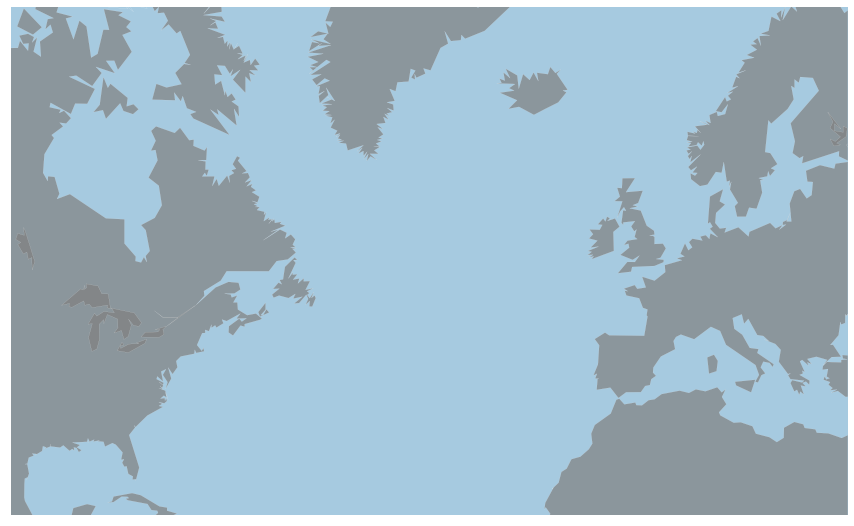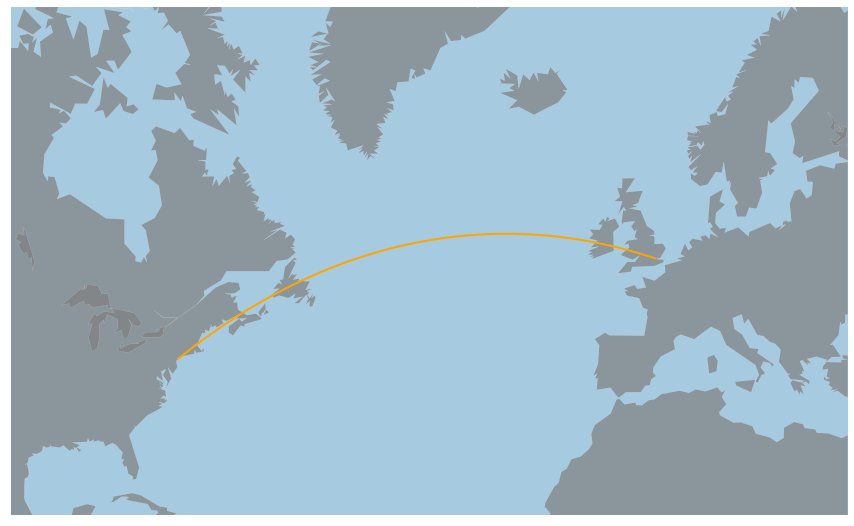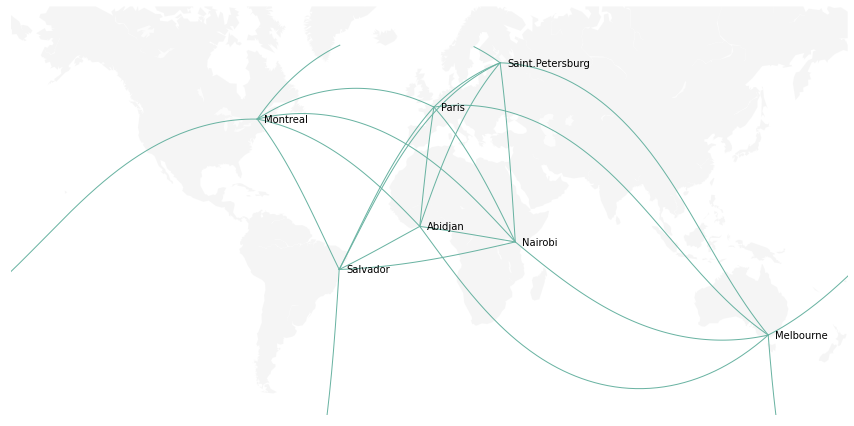## 📍 Map initialization

There are several ways to draw background maps with Python. For a complete review, visit the map section of the gallery

This example uses the `Basemap` library. Let's initialize a map of the world as explained in this post.

``````# libraries
from mpl_toolkits.basemap import Basemap
import numpy as np
import matplotlib.pyplot as plt

# Set the plot size for this notebook:
plt.rcParams["figure.figsize"]=15,12

# A basic map
m=Basemap(llcrnrlon=-100, llcrnrlat=20, urcrnrlon=30, urcrnrlat=70, projection='merc')
m.drawmapboundary(fill_color='#A6CAE0', linewidth=0)
m.fillcontinents(color='grey', alpha=0.7, lake_color='grey')
m.drawcoastlines(linewidth=0.1, color="white");``````## 🔗 Great Circle

A great circle is the intersection of the sphere and a plane that passes through the center point of the sphere. Wikipedia

Basically, a great circle shows the shortest path between 2 locations, knowing that our planet is a sphere. This path is not a straight line but an arc, which gives a much better appearance to the map.

Let's add a connection between London and New York. This is quite straightforward with `Basemap` thanks to the `drawgreatcircle()` function.

``````# Background map
m=Basemap(llcrnrlon=-100, llcrnrlat=20, urcrnrlon=30, urcrnrlat=70, projection='merc')
m.drawmapboundary(fill_color='#A6CAE0', linewidth=0)
m.fillcontinents(color='grey', alpha=0.7, lake_color='grey')
m.drawcoastlines(linewidth=0.1, color="white")

# Add a connection between new york and London
startlat = 40.78; startlon = -73.98
arrlat = 51.53; arrlon = 0.08
m.drawgreatcircle(startlon, startlat, arrlon, arrlat, linewidth=2, color='orange');

``````## 🌐 From dataframe

Let's consider a `Pandas` data frame that lists a few major cities of the world. For each city, latitude and longitude are available:

``````    # Dataframe: list of a few cities with their coordinates:
import pandas as pd
import pandas as pd
cities = {
'city': ["Paris", "Melbourne", "Saint.Petersburg", "Abidjan", "Montreal", "Nairobi", "Salvador"],
'lon': [2, 145, 30.32, -4.03, -73.57, 36.82, -38.5],
'lat': [49, -38, 59.93, 5.33, 45.52, -1.29, -12.97]
}
df = pd.DataFrame(cities, columns = ['city', 'lon', 'lat'])``````

Let's loop through this dataframe and add a connection between each pair of city. Moreover, let's use the `annotate()` function of `matplotlib` to add the city names on the map.

``````

# Background map
m=Basemap(llcrnrlon=-179, llcrnrlat=-60, urcrnrlon=179, urcrnrlat=70,  projection='merc')
m.drawmapboundary(fill_color='white', linewidth=0)
m.fillcontinents(color='#f2f2f2', alpha=0.7)
m.drawcoastlines(linewidth=0.1, color="white")

# Loop on every pair of cities to add the connection
for startIndex, startRow in df.iterrows():
for endIndex in range(startIndex, len(df.index)):
endRow = df.iloc[endIndex]
m.drawgreatcircle(startRow.lon, startRow.lat, endRow.lon, endRow.lat, linewidth=1, color='#69b3a2');